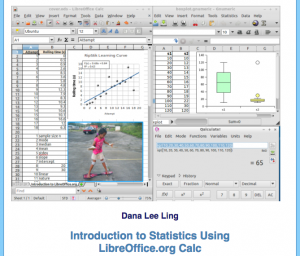# Advent Resource #20: Introduction to StatisticsDana Lee Ling is a Professor at the College of Micronesia, who – amongst other academic tasks – is teaching students to use LibreOffice Calc to make statistical calculations. To support his lessons, he has published a manual – “Introduction to Statistics Using LibreOffice Calc, AOO Calc and Gnumeric” – based on the following contents: Populations and samples, Measures of middle and spread, Visualizing data, Paired data and scatter diagrams, Probability, Probability distributions, Introduction to the normal distribution, Normal distribution and z-values, Confidence intervals for the mean, Hypothesis testing against a known population mean, and Hypothesis testing two sample means.